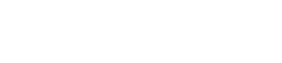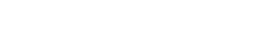# What is a Chi-Square Test?

Things don’t always come out the way that you expect in statistics. There may be a hidden bias in the choices people make or maybe the data are not created equally. To address the expected versus the unexpected, we use a special statistical test called a chi-square test (pronounced ki as in kind). It is a special type of test that deals with frequency of data instead of means like some other tests.

## Chi-Square Test is all in the Categories

I like scary movies. I once asked my students if they liked scary movies too. Being the delightful and hilarious nerd that I am, I collected the data so that I could analyze it for patterns. This is what I got.At first, it looks like boys and girls both like scary movies equally. But after a closer look, you may notice that two-thirds of the boys like scary movies compared to the half of the girls! This could represent a bias in the overall data. So, we should analyze the data to see if this pattern is statistically significant.

But there are no mean values to work with!

Since we are dealing with a purely categorical value, we need to use a test that deals with the frequency of data instead of mean values. That is what the chi-square test does. The chi-square test, like the t-test or F-test, deals with the probability of a specific set of values occurring. However, it deals with frequency counts instead of means.

### The Actual Test

Calculating the chi-square value of a data set involved measuring how often an observed outcome differs from an expected outcome. In fact, that is really all it is. Let’s see.This means that the equation will essentially give you a ratio. We’ll compare this ratio to a distribution table of critical values similar to a t-test of F-test. If the value exceeds that critical value, then there is a significant pattern in the data.

But what does that significant pattern mean?

## Proud, Strong, Independent Categories

When I talk about expected frequencies, I don’t mean a 50/50 split between boys and girls liking scary movies. Instead, we expect the frequency of students liking scary movies to be independent of gender based on probability.

The null hypothesis of a chi-square test is that the events (in this case choice of liking scary movies) are independent of gender. That means that each of the four possibilities (boy-yes, girl-yes, boy-no, girl-no) have an equal chance of occurring. For example, the possibility of a girl choosing to like scary movies (because my wife definitely does) isThis is the expected outcome. It is also slightly different from the observed outcome. This difference is what we use in the chi-square calculation. If you would like to calculate along with me, the other expected outcomes are girl-no = 31.25, boy-yes = 23.25, and boy-no = 18.75.

Our calculated chi-square value based on the formula is 7.02. Is this higher than the critical value? We will need to know the degrees of freedom in order to determine that from the table.

### Freedom is a Tricky Thing

In a chi-square test, the degrees of freedom is not just the sample size minus 1. Instead, it has to do with the size of the and number of categories that we are using. It is traditionally calculated as the (# rows – 1) x (# columns – 1) = df. In the case of our scary movies, we have 1 degree of freedom.

So, when we check the distribution table for one degree of freedom at the 0.05 level (or 5% error) level, we see that our calculated value of 7.02 is higher. This indicates that that the difference we see between the genders is significant. Liking scary movies is not independent of gender, but related to it.

One final note on degrees of freedom, sometimes there are more than one degrees of freedom because we use the chi-square test to determine if a model is independent of the data. This is an example of using chi-square to determine goodness of fit similar to the F-statistic. But that is for more complex models and for another post.

Ultimately, the chi-square test is used to determine if the frequency of data occurring is different from what is expected. It is most useful for data that is non-parametric meaning that the population does not have a normal distribution, like categorical or nominal data. You should be careful in interpreting chi-square tests because they look for independence of events instead of simple numerical difference. It takes some practice, but they are one of the most useful tests in statistics. Happy statistics!

##### Comments are closed.

Magoosh blog comment policy: To create the best experience for our readers, we will only approve comments that are relevant to the article, general enough to be helpful to other students, concise, and well-written! 😄 Due to the high volume of comments across all of our blogs, we cannot promise that all comments will receive responses from our instructors.

We highly encourage students to help each other out and respond to other students' comments if you can!

If you are a Premium Magoosh student and would like more personalized service from our instructors, you can use the Help tab on the Magoosh dashboard. Thanks!